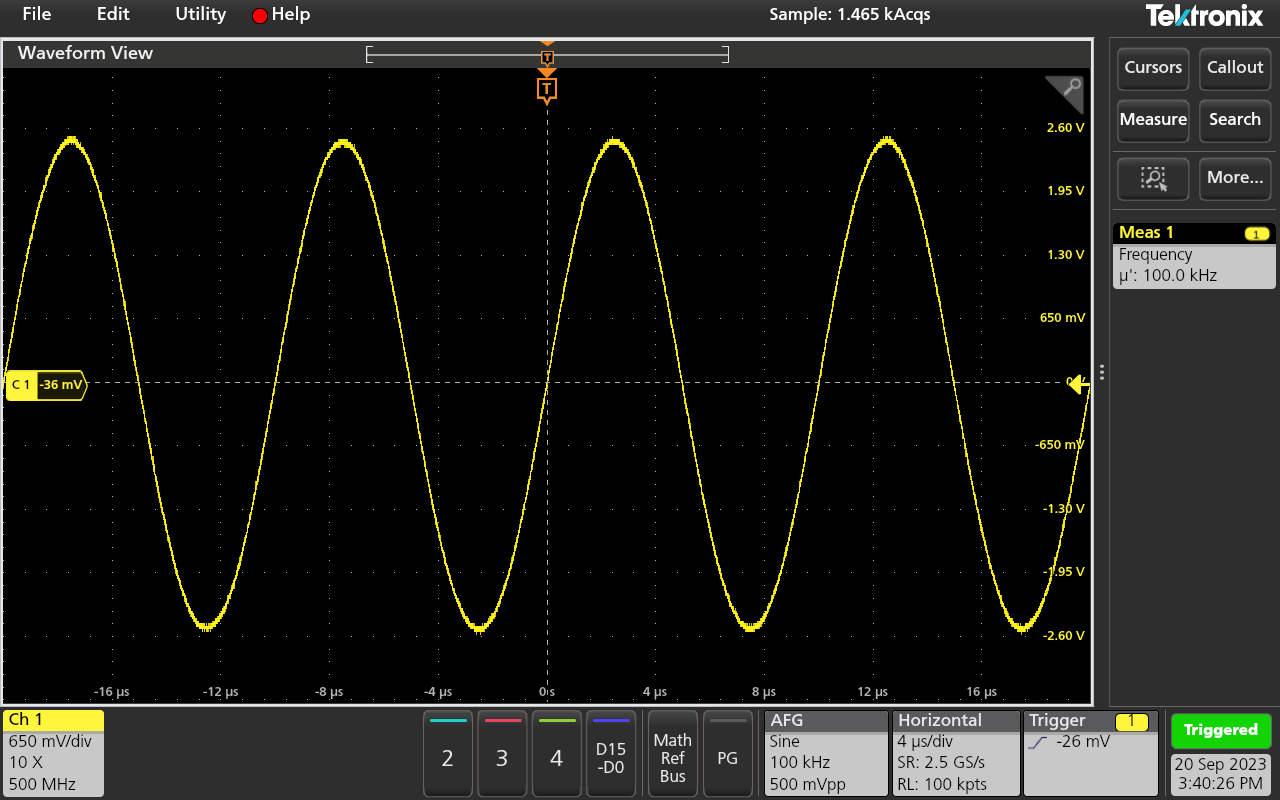# How to Measure Frequency with an Oscilloscope

Oscilloscopes measure the voltage of an electrical signal, which is then graphically represented by waveforms. However, an oscilloscope can be used to take measurements for a number of different applications – one of which is frequency. But how does an oscilloscope measure frequency, exactly? Well, there’s a straightforward answer, and then there’s a slightly more complicated answer.

On most, if not all, modern digital oscilloscopes the calculation is done for you, so you’ll be able to get a frequency reading whilst you’re measuring voltage. Simply press a couple of buttons to see the frequency of the signal you’re trying to inspect, as well as other statistics such as standard deviation and the mean frequency. However, there are ways to work out the frequency without the aid of technology.

## How to Calculate Frequency

You can measure time and frequency quite easily using the horizontal scale on your oscilloscope. If you want to ensure accuracy when taking a frequency measurement, it helps to increase the signal area on your oscilloscope display – making it bigger so you can more easily translate the waveform.

Firstly, you need to measure the time using the horizontal scale on your oscilloscope, as well as counting the number of horizontal divisions from one end of the wave to the other where it crosses the Centre Horizontal Graticule Line. Then you can multiply the number of horizontal divisions by the time/division to find the signal’s period. Once you have this, you can perform the frequency calculation: one divided by the period. Mathematically this can be represented as frequency=1/periodRead more: What does an oscilloscope measure?

## Automatic Measurements on a Digital Scope

As previously mentioned, most high-quality digital oscilloscopes will take measurements automatically, meaning that you don’t have to do the calculations. On Tektronix oscilloscopes, you can find the time measurements under the ‘measurements’ badge on the display. This will give you an accurate, live reading of the frequency, which will change depending on the signal being transmitted.

You can also access all statistic data. This way you can see the standard deviation, mean, maximum, and minimum of the frequency, providing a better idea of how the signal is reacting.### How to measure frequency using an oscilloscope

To measure frequency with an oscilloscope, follow these steps:

1. Connect the Signal: Connect the input probe of your oscilloscope to the signal source you want to measure.
2. Set Up the Oscilloscope: Turn on your oscilloscope and adjust the settings. Set the time/division (horizontal scale) to an appropriate value for the signal's frequency range.
3. Select AC Coupling: For accurate frequency measurement, select AC coupling on the channel you're using. This removes any DC offset in the signal.
4. Trigger the Oscilloscope: Set the trigger mode to "Auto" or "Normal," and adjust the trigger level as needed to stabilize the waveform on the screen.
5. Measure the Frequency: Count the number of waveform cycles that appear on the screen within one second. This value is your frequency.

#### Are there any specific settings I need to consider when measuring frequency with an oscilloscope?

Yes, make sure to set the oscilloscope to an appropriate time/division setting to ensure the waveform doesn't stretch or compress on the screen. Also, select the correct voltage range and trigger level for your signal.

#### What if the signal is complex or noisy?

If the signal is complex or noisy, you may need to use advanced features like frequency analysis tools or digital filtering on your oscilloscope to obtain accurate frequency measurements.

#### Can I measure the frequency of multiple signals simultaneously?

Most oscilloscopes allow you to measure the frequency of multiple signals using multiple channels. You can switch between channels to measure each signal individually.

#### Are there any precautions to take when using an oscilloscope for frequency measurement?

Yes, here are some precautions:

1. Ensure proper grounding to avoid electric shock.
2. Use appropriate probe settings to match the input signal's characteristics.